Courses

# Electrical Machines 4 MCQ

## 20 Questions MCQ Test Mock Test Series for SSC JE Electrical Engineering (Hindi) | Electrical Machines 4 MCQ

Description
This mock test of Electrical Machines 4 MCQ for Electrical Engineering (EE) helps you for every Electrical Engineering (EE) entrance exam. This contains 20 Multiple Choice Questions for Electrical Engineering (EE) Electrical Machines 4 MCQ (mcq) to study with solutions a complete question bank. The solved questions answers in this Electrical Machines 4 MCQ quiz give you a good mix of easy questions and tough questions. Electrical Engineering (EE) students definitely take this Electrical Machines 4 MCQ exercise for a better result in the exam. You can find other Electrical Machines 4 MCQ extra questions, long questions & short questions for Electrical Engineering (EE) on EduRev as well by searching above.
QUESTION: 1

### What happens if the magnetic neutral axis coincides with the geometric neutral axis in case of DC generator?

Solution:

In DC machines, two kinds of magnetic fluxes are present (armature flux and main field flux). The effect of armature flux on the main field flux is called as armature reaction.

EMF is induced in the armature conductors when they cut the magnetic field lines. There is an axis along which armature conductors move parallel to the flux lines hence, they do not cut the flux lines while on that plane. This axis is called MNA (Magnetic Neutral Axis).

GNA (Geometrical Neutral Axis) is perpendicular to the stator field axis.

When machine is running on no load, no current is flowing in the armature conductors and only the field winding is energized. In this case, magnetic flux lines of the field poles are uniform and symmetrical to the polar axis. The magnetic neutral axis coincides with the geometric neutral axis.

QUESTION: 2

### In D.C. generators, the polarity of the inter-pole is:

Solution:

In DC generators, the polarity of inter-poles is same as that of the polarity of the main poles ahead in a generator rotation.

In DC motors, the polarity of the inter-poles is same as that of the poles behind the main field poles.

QUESTION: 3

### Flashing the field of DC generator means:

Solution:

Residual magnetism in the generator exciter field allows the generator to build up voltage during starting. This magnetism is sometimes lost due to improper operation. Restoring this residual magnetism is possible by using a DC source. It is sometimes referred to as flashing the exciter field.

QUESTION: 4

The given figure depicts the Torque-Current characteristics of: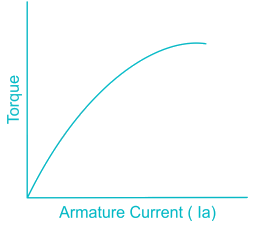Solution:

We can observe the torque current characteristics of different DC motors as shown below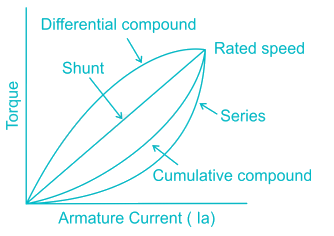QUESTION: 5

For a DC Motor for constant load torque, what will happen to the armature current if armature resistance increase?

Solution: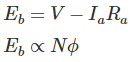If we keep an external resistance in armature circuit to increase the armature resistance,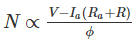At constant torque load, as armature resistance increases the back emf decreases and armature current increases.

QUESTION: 6

Which type of frame is preferred for motor in moisture surrounding?

Solution:

Electric motor with totally enclosed fan cooled (TEFC) frame that does not permit outside air to freely circulate through the interior of the motor. An external fan blows outside air over the frame of the motor to cool it. This motor is arguably the most commonly used motor in ordinary industrial environments. TEFC enclosed motors usually cost more than open motors but offer increased protection against weather, dirt and moisture.

QUESTION: 7

The sheet steel material used in transformer core has:

Solution:

The steel material used in transformer should cause less core losses.

Permeability is the measure of the ability of a material to support the formation of a magnetic field within itself. Hence, to form a strong magnetic field, the material should offer high permeability.

QUESTION: 8

A transformer is equivalent to an ideal transformer

Solution:

A transformer is equivalent to an ideal transformer with inductive coils connected in both the primary and secondary circuits.

We can represent the equivalent circuit as shown below.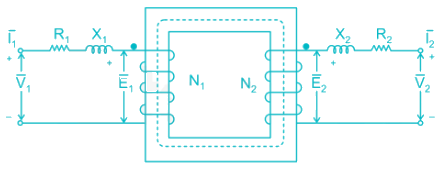QUESTION: 9

If the reciprocal of transformation ratio of a transformer is 11. The ratio of current in the secondary to primary is:

Solution:

In a transformer, transformation ratio can be defined by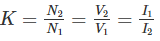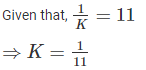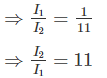QUESTION: 10

Which test is used in determining the magnetizing impedance of a transformer?

Solution:

The open circuit test on transformer is used to determine core losses in transformer and parameters of the shunt branch of the equivalent circuit of the transformer. Hence using open circuit test, we can determine the magnetizing impedance.

The short circuit test on transformer is used to determine copper loss in transformer at full load and parameters of approximate equivalent circuit of transformer.

QUESTION: 11

An alternator has: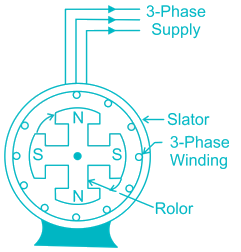This arrangement has the following advantages:

1) The stationary armature coils can be insulated easily

2) Higher peripheral speed can be achieved in the rotor

3) Cooling of the winding is more efficient

4) Only two slip rings are required to give DC supply to the field system

5) Output current can be easily supplied to the load circuit

Solution:

The field poles are placed on the stationary part of the machine. Since no commutator is required in an alternator, it is usually more convenient and advantageous to place the field winding on the rotating part (i.e., rotor) and three phase armature windings on the stationary part (i.e., stator).

QUESTION: 12

The integration of a three phase Alternator with the infinite grid requires which quantities to be same?

Solution:

For the integration of a three phase Alternator with the infinite grid we need to take care of following:

1) The voltage level of alternator and infinite grid should be same

2) The operating frequency of alternator and infinite grid should be same

3) The phase sequence of alternator and infinite grid should be same

QUESTION: 13

As compared to D.O.L. starter, star delta starter operates at:

Solution:

In case of star delta starter, the stator terminals are first connected in star. In star connection, phase voltage is 0.577 times the line voltage. If connected in delta, the line and phase voltage are same.

Now, current through each phase winding is equal to Phase Voltage/impedance of the winding. So, if phase Voltage is reduced, the starting current can be reduced because the impedance remains constant. That's why the motor is started in star connection where voltage reduces by √3 times or 0.577 times thus reducing the starting current.

Hence as compared to D.O.L. starter, star delta starter operates at 58% of full voltage.

QUESTION: 14

A 3-phase induction motor is running on a constant load. If the fuse in one phase blows off the

Solution:

When a three-phase motor loses one of the phase supply voltage then it is called single phasing. Single phasing is a case when any one phase out of the three phases fails.

Since one of the phases is now disconnected, current through other two phases will increase to produce the desired torque. The motor will run but would not be able to drive rated load. The uneven torque results in abnormal noise and vibration in motor.

QUESTION: 15

The magnitude of armature current in synchronous motor operation:

Solution:

In synchronous motor operation, the magnitude of armature current has large value and lags for low excitation. We can observe this by V curves given below.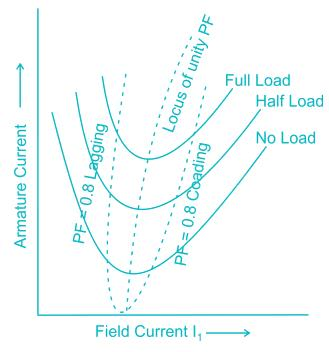QUESTION: 16

Which motor is generally used in rolling mills, paper and cement industries?

Solution:

The important characteristics of the synchronous motor is its constant speed irrespective of the load conditions and variable power factor operation.

Due to constant speed characteristics, it is used in machine tools, motor generator sets, synchronous clocks, stroboscopic devices, timing devices, belt driven reciprocating compressors, fans and blowers, centrifugal pumps, vacuum pumps, pulp grinders, textile mills, paper mills line shafts, rolling mills, cement mills etc.

QUESTION: 17

Speed control of single-phase series motor (AC) can be done by:

Solution:

Speed control of single-phase series motor (universal motor) is best obtained by solid-state devices by varying the voltage. Since the speed of these is not limited by the supply frequency and may be as high as 20000 rpm. They are most suitable for applications requiring high speeds.

QUESTION: 18

As per double field revolving theory with slip denoted by:

Solution:

According to double field revolving theory, we can resolve any alternating quantity into two components. Each component has a magnitude equal to the half of the maximum magnitude of the alternating quantity, and both these components rotate in the opposite direction to each other.

For example, a flux, φ can be resolved into two components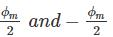Each of these components rotates in the opposite direction i.e. if one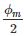is rotating in clockwise direction then the otherrotates in anticlockwise direction.

In single phase induction motor, let us call these two components of flux as forwarding component of flux ϕf and the backward component of flux ϕb.

The resultant of these two components of flux at any instant of time gives the value of instantaneous stator flux at that particular instant.

ϕr = ϕf + ϕb

The forward flux has a slip of s and backward flux have a slip of 2-s.

QUESTION: 19

In a single-phase induction motor the stator winding produces a flux which is:

Solution:

Single phase induction motor has distributed stator winding and has squirrel cage rotor. When fed from a single-phase supply, its stator winding produces a flux which is only alternating i.e. one which alternates along one space axis only.

QUESTION: 20

A capacitor-start single phase induction motor is switched on to supply with its capacitor and replaced by an inductor of equivalent reactance value. It will:

Solution:

In 1-phase induction motors, we are unable to get starting torque. So, we mostly use permanent capacitor split phase starting method. Its main aim is to split the single-phase supply into 2 phases, having time displacement of 90 degrees, also mechanically the 2 windings are placed 90 degrees in space. It gives rotating magnetic field which produces a starting torque & makes it a self-starting motor.

If inductor is used, the motor would not start even if having same reactance. Since coils are inductive so a capacitor or some phase splitting is necessary.